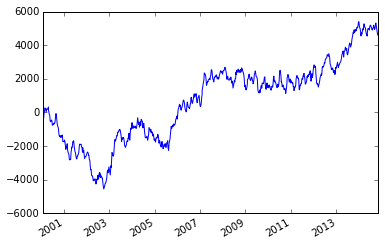# Welcome to an example Binder¶

This notebook uses a Python environment with a few libraries, including dask, all of which were specificied using a conda environment.yml file. To demo the environment, we'll show a simplified example of using dask to analyze time series data, adapted from Matthew Rocklin's excellent repo of dask examples — check out that repo for the full version (and many other examples).

## Setup plotting¶

In :
%matplotlib inline


## Turn on a global progress bar¶

In :
from dask.diagnostics import ProgressBar

In :
progress_bar = ProgressBar()
progress_bar.register()


## Generate fake data¶

In :
import dask.dataframe as dd

In :
df = dd.demo.make_timeseries(start='2000', end='2015', dtypes={'A': float, 'B': int},
freq='5s', partition_freq='3M', seed=1234)


## Compute and plot a cumulative sum¶

In :
df.A.cumsum().resample('1w').mean().compute().plot();

[########################################] | 100% Completed | 16.5s